## Example Questions

← Previous 1

### Example Question #1 : How To Find The Length Of The Diameter

If the area of a circle is four times larger than the circumference of that same circle, what is the diameter of the circle?

16

2

8

4

32

16

Explanation:

Set the area of the circle equal to four times the circumference πr2 = 4(2πr).

Cross out both π symbols and one r on each side leaves you with r = 4(2) so r = 8 and therefore = 16.

### Example Question #1 : How To Find The Length Of The Diameter

The perimeter of a circle is 36 π.  What is the diameter of the circle?

36

3

72

6

18

36

Explanation:

The perimeter of a circle = 2 πr = πd

Therefore d = 36

### Example Question #131 : Geometry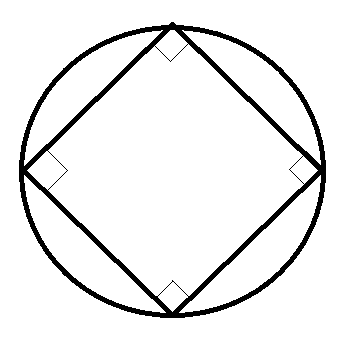If the area of the circle touching the square in the picture above is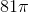, what is the closest value to the area of the square?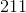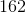Explanation:

Obtain the radius of the circle from the area.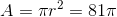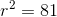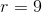Split the square up into 4 triangles by connecting opposite corners. These triangles will have a right angle at the center of the square, formed by two radii of the circle, and two 45-degree angles at the square's corners. Because you have a 45-45-90 triangle, you can calculate the sides of the triangles to be,, and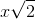. The radii of the circle (from the center to the corners of the square) will be 9. The hypotenuse (side of the square) must be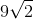.

The area of the square is then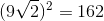.

### Example Question #1 : How To Find The Length Of The Diameter

Two legs of a right triangle measure 3 and 4, respectively. What is the area of the circle that circumscribes the triangle?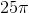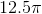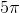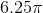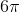Explanation:

For the circle to contain all 3 vertices, the hypotenuse must be the diameter of the circle. The hypotenuse, and therefore the diameter, is 5, since this must be a 3-4-5 right triangle.

The equation for the area of a circle is A = πr2.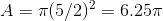### Example Question #1 : How To Find The Length Of The Diameter

The circumference of the circle is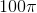.  What is the diameter?Explanation:

Write the formula for the circumference.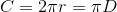Substitute the circumference.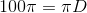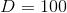### Example Question #2 : How To Find The Length Of The Diameter

Find the diameter of a circle whose area is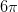.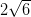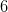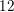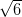Explanation:

To solve, simply use the formula for the area of a circle to find the radius, and then multiply it by 2 to find the diameter. Thus,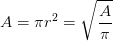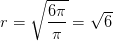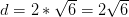### Example Question #2 : How To Find The Length Of The Diameter

Find the length of the diameter given radius of 1.Explanation:

To solve, simply use the formula for the diameter of a circle. Thus,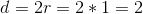### Example Question #4 : How To Find The Length Of The Diameter

Find the length of the diameter given the radius is 5.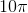Explanation:

To solve, simply use the formula for the diameter of a circle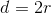where r is 5. Thus,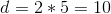Remember, the diameter is the longest distance across a circle, and since the radius is 5, you can simply double that. Thus, the answer is 10.

### Example Question #5 : How To Find The Length Of The Diameter

Find the diameter of a circle given the radius is 6.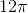Explanation:

To solve, simply use the formula for the diameter of circle.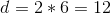Remember, since the diameter is distance between two points on opposite sides of the circle, you simply double the radius. No pi is involved in diameter, only in circumference, area, etc.

### Example Question #6 : How To Find The Length Of The Diameter

The circumference of a given circle is half of its area. What is the diameter of the circle?Explanation:

Remember that the circumference of a circle is given byand the area is given byIf the circumference of this circle if one half its area, then we can say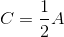. Or,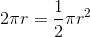We can solve this equation for r like so: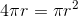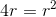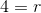Since the diameter of a circle is twice its radius, then the diameter of this circle is 8.

To check your answer, plug in r=4 into the circumference and area formulas. You will see that the area of this circle is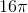and its circumference is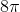, which is exactly half its area.

← Previous 1

### All SAT Math Resources